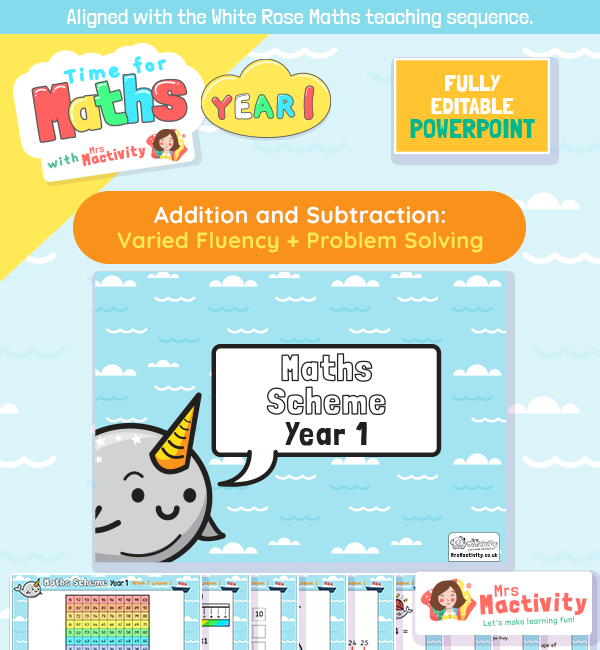# Varied Fluency Addition and Subtraction Within 20 Lesson Presentation## White Rose Maths varied fluency resources

Aligned with the White Rose Maths scheme of work, this Varied Fluency Addition and Subtraction Within 20 Lesson Presentation is fully editable, and is designed for the year 1 maths curriculum covering the following maths objectives for the spring term:

• Count forwards and backwards from any given number to 100
• Add and subtract number bonds to 20
• Count in 2s, 5s and 10s

Aligned with the order of teaching of the White Rose Maths scheme of work, use this to help your children get to grips with subtraction. Includes maths continuous provision ideas and non-worksheet maths learning too.

Explore our maths mastery resources.

Explore our year 1 maths resources.

Explore our addition and subtraction resources.

Free